# Stefan problem

A problem that arises when studying physical processes related to phase transformation of matter. The simplest two-phase Stefan problem is formulated in thermo-physical terms as follows (, ): Find the distribution of the temperature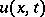and the law of motion of the dividing boundary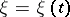(for example, the boundary "ice-water" in freezing water) from the equation of heat conductivity: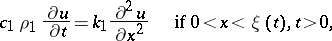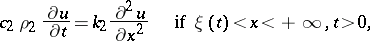with the boundary condition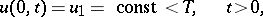the initial condition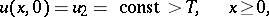and the conditions on the freezing boundary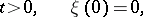where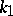andare the coefficients of heat conductivity,and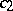are the specific heats,and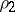are the densities of the solid and the corresponding liquid phase,is the latent heat of melting per unit of mass, andis the temperature of freezing. This problem has a self-similar solution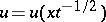,,.

A sufficiently-general formulation of the Stefan problem in the three-dimensional spatial case reduces to a boundary value problem for a quasi-linear parabolic equation of the second order with piecewise-continuous coefficients having discontinuities of the first kind on previously-unknown but sought-for surfaces, on which the value of the required function is defined, and which also satisfy the differential Stefan condition. The existence and uniqueness of classical and generalized solutions of the Stefan problem have been studied (); for methods of approximate solution of the Stefan problem, see , , .

One of the first to study this type of problem was J. Stefan .